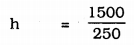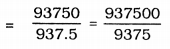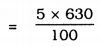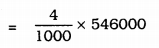# KSEEB Solutions for Class 9 Maths Chapter 13 Surface Area and Volumes Ex 13.1

## Karnataka Board Class 9 Maths Chapter 13 Surface Area and Volumes Ex 13.1

Question 1.
A plastic box 1.5 m long, 1.25 m wide and 65 cm deep is to be made. It is opened at the top. Ignoring the thickness of the plastic sheet, determine :
(i) The area of the sheet required for making the box.
(ii) The cost of the sheet for it, if a sheet measuring 1 m2 costs Rs. 20.
Solution:
(i) Length of plastic box, l = 1.5 m
height, h = 65 cm = 0.65 m.
= (1.5 × 1.25) + (2 × 1.25 × 0.65) + 2(1.5)(0.65)
= 1.875 + 1.625 + 1.950
A= 5.45 m2.
(ii) Cost of 1 sq. m sheet is Rs. 20
cost of 5.45 sq.m, the sheet is = 5.45 × 20 = Rs. 109.

Question 2.
The length, breadth and height of a room are 5 m, 4 m and 3 m respectively. Find the cost of whitewashing the walls of the room and the ceiling at the rate of Rs. 7.50 per m2.
Solution:
Length of room, l = 5 m
height, h = 3 m
White washing the room and the ceiling except floor to be made.
∴ Area, A = lb + 2bh + 21h
= lb + 2h(b + l)
= (5 × 4) + 2 × 3(4 + 5)
= 20 + 6(9)
= 20 + 54
= 74 m2.
Cost of white washing for 1 sq.m. is Rs. 7.50
∴ Cost of white washing 74 sq. m. is ?
= 74 × 7.50
= Rs. 555.

Question 3.
The floor of a rectangular hall has a perimeter 250 m. If the cost of painting the four walls at the rate of Rs. 10 per m2 is Rs. 15000, find the height of the hall. [Hint: Area of the four walls = Lateral surface area.]
Solution:
Perimeter of a rectangle room = 250 m
= 2(l + b)
Cost of Painting = Rs. 15000
For Rs. 10, 1 sq.m.
For Rs.15000 … ? $$=\frac{15000}{10}$$
= 1500m2
Area of four walls = Lateral surface area
Lateral surface area of rectangle = 1500 m2
2h(l + b) = 1500
h × 2(l + b) = 1500
h × 250 = 1500∴ h = 6 m

Question 4.
The paint in a certain container is sufficient to paint an area equal to 9.375 m2. How many bricks of dimensions 22.5 cm × 10 cm × 7.5 cm can be painted out of this container?
Solution:
Length of Brick l = 22.5 cm
height, h = 7.5 cm.
Area to be painted, A = 9.375 m2
= 93750 cm2
Total surface area of brick, A
A= 2(lb + bh + lh)
= 2[22 × 10 + 10 × 7.5 + 22.5 × 7.5]
= 2[468.75]
= 937.5 cm2.
Number of bricks to be painted, For 937.5 cm2, 1 brick is required For 93750 cm2 …??…= 100
∴ 100 bricks have to be painted.

Question 5.
A cubical box has each edge 10 cm and another cuboidal box is 12.5 cm long, 10 cm wide and 8 cm high.
(i) Which box has the greater lateral surface area and by how much?
(ii) Which box has the smaller total surface area and by how much?
Solution:
Each edge of cubical box, a = 10 cm.
(i) Curved Surface Area = 4a2 = 4(10)2
= 4 × 100
= 400 cm2.
Length of cuboid box, l = 12.5 cm.
b = 10 cm
h = 8 cm.
Curved Surface area of Cuboid, A = 2h (l+ b)
A = 2 × 8(12.5 + 10)
= 16 × 22.5
= 360 cm2.
∴ 400 – 360 = 40 cm2.
L.S.A. of cuboid box is 40 cm2 greater than rectangular cuboid.

(ii) Total surface area of cube (T.S.A.) = 6a2
= 6(10)2
= 6 × 100
= 600 cm2.
T.S.A. of cuboid = 2(Ib + lh + bh)
= 2[ 12.5 × 10 + 12.5 × 8 + 10 × 8]
= 2 (125 + 100 + 80)
= 2 × 305
= 610 cm2.
Here, cuboid box’s T.S.A. is more than T.S.A. of cubical box, i.e,
610 – 600 = 10 cm2 more.

Question 6.
A small indoor greenhouse (herbarium) is made entirely of glass panes (including base) held together with tape. It is 30 cm long, 25 cm wide and 25 cm high.
(i) What is the area of the glass?
(ii) How much of tape is needed for all the 12 edges?
Solution:
(i) Length of Herbarium, l = 30 cm,
height, h = 25 cm.
∴ TSA of Herbarium = 2 (lb + bh + Hi)
= 2 [30 × 25 + 25 × 25 + 30 × 25]
= 2 [750 + 625 + 750]
= 2 (2125)
= 4250 cm2.

(ii) Tape required for all 12 edges means, it has 4 length, 4 breadth and 4 heights.
∴ Length of tape required = 4 (l + b + h)
= 4 (30 + 25 + 25)
= 4 × 80
= 320 cm.

Question 7.
Shanti Sweets Stall was placing an order for making cardboard boxes for packing their sweets. Two sizes of boxes were required. The bigger of dimensions 25 cm × 20 cm × 5 cm and the smaller of dimensions 15 cm × 12 cm × 5 cm. For all the overlaps, 5% of the total surface area is required extra. If the cost of the cardboard is Rs. 4 for 1000 cm2, find the cost of cardboard required for supplying 250 boxes of each kind.
Solution:
Measurement of big box, l = 25 cm.
b = 20 cm.
h = 5 cm.
∴ TSA of eacxh big box = 2(lb + bh + lh)
= 2 [25 × 20 + 20 × 5 + 25 × 5]
= 2 [500 + 100 + 120]
= 2 (725)
T.S.A. =1450 cm2.
For all the overlaps 5% of the total surface area is required extra.= 72.5 cm2.
∴ Total surface area of big box, TSA = 1450 + 72.5 = 1522.5 cm2.
Area required for 1 box is 1522.5 cm2 Area required for 25 boxes … ?…
= 1522.5 × 250
= 380625 cm2.
Dimension of small box, l = 15 cm.
b = 12 cm.
h = 5 cm.
∴ TSA of each small box = 2(lb + bh + lh)
= 2 [15 × 12 + 12 × 5 + 15 × 5]
= 2 [180 + 60 + 75]
= 2 × 315
= 630 cm2.
More area to overlap cardboard is 5% more.
For 100 cm2, 5 cm2
For 630 cm2, …?…= 31.5 cm2.
∴ T.S.A.= 630 + 31.5= 661.5 cm2.
TSA required for 1 box is 661.5 cm2
TSA required for 250 boxes …?…
= 661.5 × 250
= 165375 cm2.
∴ To prepare 500 boxes, TSA = 380625 + 165375
= 546000 cm2.
Cost of 1000 sq.cm, of cardboard is Rs.4 Cost of 546000 sq.cm of cardboard is ?= Rs. 2184

Question 8.
Parveen wanted to make a temporary shelter for her car, by making a box-like structure with a tarpaulin that covers all the four sides and the top of the car (with the front face as a flap which can be rolled up). Assuming that the stitching margins are very small, and therefore negligible, how many tarpaulins would be required to make the shelter of height 2.5 m, with base dimensions 4m × 3m?
Solution:
Measurement of the roof, l = 4 m.
b = 3 m.
h = 2.5 m.
∴ Area of Tarpaulin = 2 (lb + bh) + lh
= 2 [4 × 2.5 + 3 × 2.5] +4 × 3
= 2 [10 + 7.5] + 12
= 2 × 17.5 + 12
= 35 + 12
= 47 m2.

##### KSEEB Solutions for Class 9 Maths
error: Content is protected !!